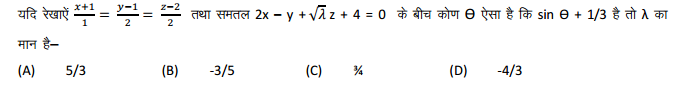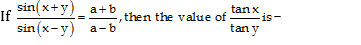## Online Quiz#### Courses

Q 1)

Which is having least mpt. :-

A) Ba

B) Ca

C) Mg

D) Be

E)

Q 2)

In the Rigvedic period, after the king the next most Important functionary of the State was the
(a) Senani
(b) Revenue collector
(c) Magician-doctor
(d) Purohita

A) a

B) b

C) c

D) d

Q 3)

Number of students in B.Sc. is approximately what percentage of the number of students in B.Ed.?

A) 167

B) 162

C) 157

D) 153

E) 150

Q 4)A) 1

B) 2

C) 3

D) 4

Q 5)

The seller lowered the prices.
• A. The prices lowered the seller.
• B. The prices were lowered by the seller.
• C. Down went the price.
• D. The prices lowered by the seller.

A) a

B) b

C) c

D) d

Q 6)

What is the ratio of total females in City A and literate females in the same city?

A) 2 : 1

B) 1 : 2

C) 3 : 2

D) 2 : 3

E) 1 : 1

Q 7)

The percentage of time, which he spends in school, is

A) 38%

B) 30%

C) 40%

D) 25%

E) none of these

Q 8)

What is the financial limit Under MUDRA's Kishor scheme?
(a) Rs. 50,000 to Rs. 10 lakh
(b) Rs. 50,000 to Rs. 3 lakh
(c) Rs. 50,000 to Rs. 1 lakh
(d) Rs. 50,000 to Rs. 2 lakh
(e) Rs. 50,000 to Rs. 5 lakh

A) a

B) b

C) c

D) d

E)

Q 9)

The radius of a circle is twice the side of a square of area 196 sq.cm. Length of a rectangle is twice diameter of the circle. What is the perimeter of the rectangle if its breadth is haft the length of the rectangle?

A) 244 cm

B) 168 cm

C) 336 cm

D) Cannot be determined

E) None of these

Q 10)A)

B)

C)

D)